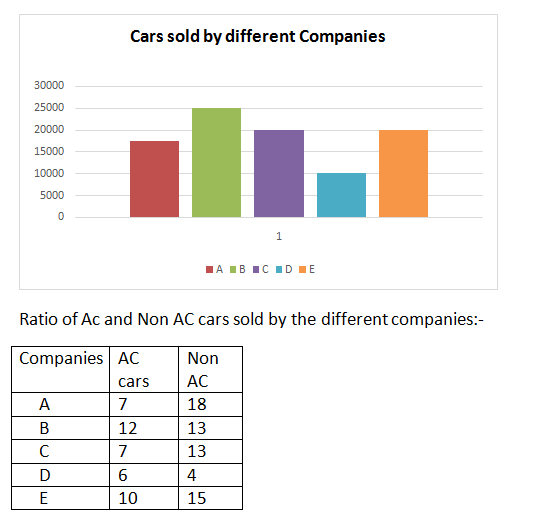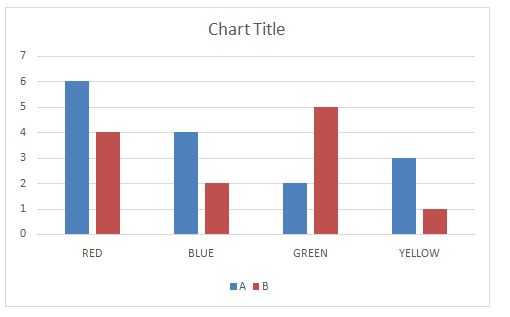# Quantitative Aptitude Questions (Data Interpretation) for SBI Clerk 2018 Day-18

## Quantitative Aptitude Questions (Data Interpretation) for SBI Clerk 2018 Day-18:

Dear Readers, SBI is conducting Online preliminary Examination for the recruitment of Clerical Cadre. preliminary Examination of SBI Clerk was scheduled from March 2018. To enrich your preparation here we have providing new series of Data Interpretation – Quantitative Aptitude Questions. Candidates those who are appearing in SBI Clerk Prelims Exam can practice these Quantitative Aptitude average questions daily and make your preparation effective.

Click “Start Quiz” to attend these Questions and view Solutions

## Daily Practice Test Schedule | Good Luck

 Topic Daily Publishing Time Daily News Papers & Editorials 8.00 AM Current Affairs Quiz 9.00 AM Logical Reasoning 10.00 AM Quantitative Aptitude “20-20” 11.00 AM Vocabulary (Based on The Hindu) 12.00 PM Static GK Quiz 1.00 PM English Language “20-20” 2.00 PM Banking Awareness Quiz 3.00 PM Reasoning Puzzles & Seating 4.00 PM Daily Current Affairs Updates 5.00 PM Data Interpretation / Application Sums (Topic Wise) 6.00 PM Reasoning Ability “20-20” 7.00 PM English Language (New Pattern Questions) 8.00 PM General / Financial Awareness Quiz 9.00 PM

Directions (Q. 1-5) Study the following information carefully and answer the given questions:

Data related to sales of car by different cars in the year 2006:-

Ratio of Ac and Non AC cars sold by the different companies:-1. Find the average no of Non AC car sold by Company A,C,D,E.
1. 9700
2. 10400
3. 8300
4. 4500
5. None of these
1. The number of Ac car sold by company B in 2006 to men and women is in ratio of 7:5, then find the difference between the Ac car purchased by men and women?
1. 4000
2. 3000
3. 2500
4. 2000
5. None of these
1. The sale of AC car in 2007 by company D decreased by 30 % as compared to previous year then find the percentage by which the sale of non AC car increased?
1. 20
2. 15
3. 12
4. Can’t be determined
5. none of these
1. The no of AC car sold by company F in 2006 is 30 % less than the AC car sold by company C then find the difference between the Non AC car sold by company A and AC car sold by F?
1. 6300
2. 2500
3. 7700
4. 6777
5. None of these
1. If company B exported 60% of its AC car then AC car sold by company B in India is what percentage less than the non AC car sold by company E?
1. 30
2. 35
3. 60
4. 24
5. None of these

Directions (Q. 6-10) Study the following information carefully and answer the given questions:

Number of different balls in two bags A and B:-1. If two balls are picked at random from Bag A then what is the probability that both balls are red?
1. 1/7
2. 3/7
3. 2/7
4. 3/14
5. None of these

7. If three balls are picked at random from Bag B, what is the probability that two are red and one is green?

1. 3/25
2. 3/22
3. 4/23
4. 5/24
5. None of these
1. If four balls are picked at random from Bag A what is the probability that one is green, two are blue and one is red?
1. 23/455
2. 3/910
3. 24/455
4. 1/66
5. None of these
1. If two balls are picked at random from Bag B, what is the probability that both are blue?
1. 1/66
2. 3/74
3. 6/77
4. 4/27
5. None of these
1. If two balls are picked at random from Bag A, what is the probability that either both are green or both are yellow?
1. 2/105
2. 6/105
3. 9/51
4. 4/105
5. None of these

Directions (Q. 1-5)

No of Non AC car sold by different cars

A= 18/25*17500= 12600

C= 13/20*20000=13000

D= 4/10*10000= 4000

E= 15/25* 20000= 12000

Total cars sold by the companies= 41600

Average = 41600/4= 10400 cars.

AC car sold by company B in 2006= 12/25*25000= 12000

AC cars purchased by men= 12000*7/12=7000

AC cars purchased by women= 5000

Difference = 7000-5000= 2000

Can’t be determined

AC car sold by C= 7/20* 20000= 7000

AC car sold by F= 7000*70/100= 4900

Non AC car sold by A= 17500*18/25= 12600

Required difference= 12600 -4900= 7700 cars

AC Cars sold by B = 25000*12/25= 12000

AC cars exported by B= 12000*60/100= 7200

AC cars sold by B in India- 12000-7200= 4800

Non AC cars sold by E= 15/25*20000= 12000

Required Percentage= 12000-4800/12000*100=60%

Directions (Q. 6-10)

Total number of balls in Bag A

= 6+4+2+3=15

Ways of selection of two red balls

=n(E)= 6C2

Ways of selection of two balls

= n(S) =15C2

So required probability= 6C2 /15C2

= 6*5/15*14

= 1/7

Total number of balls =12

= 12C3

Ways to pick 2 red balls= 4C2= 6

Ways to pick one green balls= 5C1= 5

Required probability= 6*5/220= 3/22

Ways of selection of 4 balls = 15C4

Ways of selection of one green ball= 2C1

Ways of selection two blue balls= 4C2

Ways of selection of one red ball= 6C1

Required probability = 24/455

Total balls =12

Total outcomes =12C2

Favorable outcomes = 2C2 =1

Required Probability= 1/66

Total Ways to select two green balls= 2C2

Total Ways to select two yellow balls= 3C2

Probability of both green balls= 1 / 15C2

Probability of both yellow balls= 3 / 15C2

Required probability = 1 / 15C2 + 3 / 15C2 = 4/105.